Search by Topic

Resources tagged with Cubes & cuboids similar to Little Boxes:

Filter by: Content type:
Age range:
Challenge level:

There are 52 results

Broad Topics > 3D Geometry, Shape and Space > Cubes & cuboids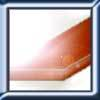Little Boxes

Age 7 to 11 Challenge Level:

How many different cuboids can you make when you use four CDs or DVDs? How about using five, then six?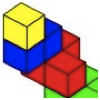Four Layers

Age 5 to 11 Challenge Level:

Can you create more models that follow these rules?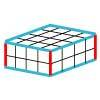Making Cuboids

Age 7 to 11 Challenge Level:

Let's say you can only use two different lengths - 2 units and 4 units. Using just these 2 lengths as the edges how many different cuboids can you make?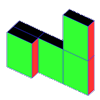Cereal Packets

Age 7 to 11 Challenge Level:

How can you put five cereal packets together to make different shapes if you must put them face-to-face?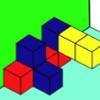Three Sets of Cubes, Two Surfaces

Age 7 to 11 Challenge Level:

How many models can you find which obey these rules?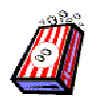Age 7 to 11 Challenge Level:

We went to the cinema and decided to buy some bags of popcorn so we asked about the prices. Investigate how much popcorn each bag holds so find out which we might have bought.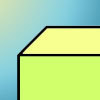Cuboid-in-a-box

Age 7 to 11 Challenge Level:

What is the smallest cuboid that you can put in this box so that you cannot fit another that's the same into it?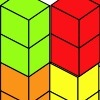Triple Cubes

Age 5 to 11 Challenge Level:

This challenge involves eight three-cube models made from interlocking cubes. Investigate different ways of putting the models together then compare your constructions.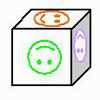Age 7 to 11 Challenge Level:

Make a cube with three strips of paper. Colour three faces or use the numbers 1 to 6 to make a die.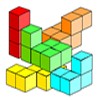Three Cubed

Age 7 to 11 Challenge Level:

Can you make a 3x3 cube with these shapes made from small cubes?Construct-o-straws

Age 7 to 11 Challenge Level:

Make a cube out of straws and have a go at this practical challenge.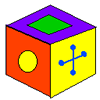A Puzzling Cube

Age 7 to 11 Challenge Level:

Here are the six faces of a cube - in no particular order. Here are three views of the cube. Can you deduce where the faces are in relation to each other and record them on the net of this cube?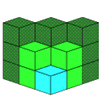Age 7 to 11 Challenge Level:

If you had 36 cubes, what different cuboids could you make?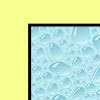All Wrapped Up

Age 7 to 11 Challenge Level:

What is the largest cuboid you can wrap in an A3 sheet of paper?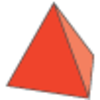Next Size Up

Age 7 to 11 Challenge Level:

The challenge for you is to make a string of six (or more!) graded cubes.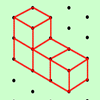The Third Dimension

Age 5 to 11 Challenge Level:

Here are four cubes joined together. How many other arrangements of four cubes can you find? Can you draw them on dotty paper?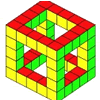Holes

Age 5 to 11 Challenge Level:

I've made some cubes and some cubes with holes in. This challenge invites you to explore the difference in the number of small cubes I've used. Can you see any patterns?Dicey

Age 7 to 11 Challenge Level:

A game has a special dice with a colour spot on each face. These three pictures show different views of the same dice. What colour is opposite blue?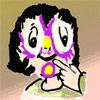Painted Faces

Age 7 to 11 Challenge Level:

Imagine a 3 by 3 by 3 cube made of 9 small cubes. Each face of the large cube is painted a different colour. How many small cubes will have two painted faces? Where are they?Cubes

Age 7 to 11 Challenge Level:

How many faces can you see when you arrange these three cubes in different ways?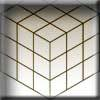Christmas Presents

Age 7 to 11 Challenge Level:

We need to wrap up this cube-shaped present, remembering that we can have no overlaps. What shapes can you find to use?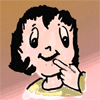Cube Drilling

Age 7 to 11 Challenge Level:

Imagine a 4 by 4 by 4 cube. If you and a friend drill holes in some of the small cubes in the ways described, how many will not have holes drilled through them?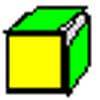Green Cube, Yellow Cube

Age 7 to 11 Challenge Level:

How can you paint the faces of these eight cubes so they can be put together to make a 2 x 2 x 2 cube that is green all over AND a 2 x 2 x 2 cube that is yellow all over?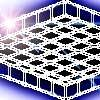More Christmas Boxes

Age 7 to 11 Challenge Level:

What size square should you cut out of each corner of a 10 x 10 grid to make the box that would hold the greatest number of cubes?Painting Cubes

Age 11 to 14 Challenge Level:

Imagine you have six different colours of paint. You paint a cube using a different colour for each of the six faces. How many different cubes can be painted using the same set of six colours?Counting Triangles

Age 11 to 14 Challenge Level:

Triangles are formed by joining the vertices of a skeletal cube. How many different types of triangle are there? How many triangles altogether?Take Ten

Age 11 to 14 Challenge Level:

Is it possible to remove ten unit cubes from a 3 by 3 by 3 cube so that the surface area of the remaining solid is the same as the surface area of the original?Cuboids

Age 11 to 14 Challenge Level:

Find a cuboid (with edges of integer values) that has a surface area of exactly 100 square units. Is there more than one? Can you find them all?Dice, Routes and Pathways

Age 5 to 14

This article for teachers discusses examples of problems in which there is no obvious method but in which children can be encouraged to think deeply about the context and extend their ability to. . . .Thinking 3D

Age 7 to 14

How can we as teachers begin to introduce 3D ideas to young children? Where do they start? How can we lay the foundations for a later enthusiasm for working in three dimensions?All in the Mind

Age 11 to 14 Challenge Level:

Imagine you are suspending a cube from one vertex and allowing it to hang freely. What shape does the surface of the water make around the cube?Troublesome Dice

Age 11 to 14 Challenge Level:

When dice land edge-up, we usually roll again. But what if we didn't...?Icosian Game

Age 11 to 14 Challenge Level:

This problem is about investigating whether it is possible to start at one vertex of a platonic solid and visit every other vertex once only returning to the vertex you started at.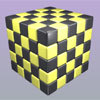Drilling Many Cubes

Age 7 to 14 Challenge Level:

A useful visualising exercise which offers opportunities for discussion and generalising, and which could be used for thinking about the formulae needed for generating the results on a spreadsheet.How Many Dice?

Age 11 to 14 Challenge Level:

A standard die has the numbers 1, 2 and 3 are opposite 6, 5 and 4 respectively so that opposite faces add to 7? If you make standard dice by writing 1, 2, 3, 4, 5, 6 on blank cubes you will find. . . .Tic Tac Toe

Age 11 to 14 Challenge Level:

In the game of Noughts and Crosses there are 8 distinct winning lines. How many distinct winning lines are there in a game played on a 3 by 3 by 3 board, with 27 cells?Nine Colours

Age 11 to 16 Challenge Level:

Can you use small coloured cubes to make a 3 by 3 by 3 cube so that each face of the bigger cube contains one of each colour?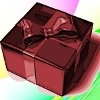Christmas Boxes

Age 11 to 14 Challenge Level:

Find all the ways to cut out a 'net' of six squares that can be folded into a cube.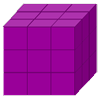Cubist Cuts

Age 11 to 14 Challenge Level:

A 3x3x3 cube may be reduced to unit cubes in six saw cuts. If after every cut you can rearrange the pieces before cutting straight through, can you do it in fewer?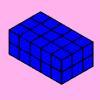Changing Areas, Changing Volumes

Age 11 to 14 Challenge Level:

How can you change the surface area of a cuboid but keep its volume the same? How can you change the volume but keep the surface area the same?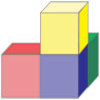Castles in the Middle

Age 7 to 14 Challenge Level:

This task depends on groups working collaboratively, discussing and reasoning to agree a final product.Always, Sometimes or Never? Shape

Age 7 to 11 Challenge Level:

Are these statements always true, sometimes true or never true?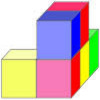The Solid

Age 11 to 16 Challenge Level:

A task which depends on members of the group working collaboratively to reach a single goal.Sending a Parcel

Age 11 to 14 Challenge Level:

What is the greatest volume you can get for a rectangular (cuboid) parcel if the maximum combined length and girth are 2 metres?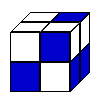Cubic Conundrum

Age 7 to 16 Challenge Level:

Which of the following cubes can be made from these nets?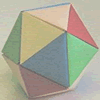Paper Folding - Models of the Platonic Solids

Age 7 to 16

A description of how to make the five Platonic solids out of paper.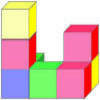Solids

Age 11 to 16 Challenge Level:

A task which depends on members of the group working collaboratively to reach a single goal.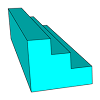Which Solid?

Age 7 to 16 Challenge Level:

This task develops spatial reasoning skills. By framing and asking questions a member of the team has to find out what mathematical object they have chosen.Marbles in a Box

Age 11 to 16 Challenge Level:

How many winning lines can you make in a three-dimensional version of noughts and crosses?Plutarch's Boxes

Age 11 to 14 Challenge Level:

According to Plutarch, the Greeks found all the rectangles with integer sides, whose areas are equal to their perimeters. Can you find them? What rectangular boxes, with integer sides, have. . . .